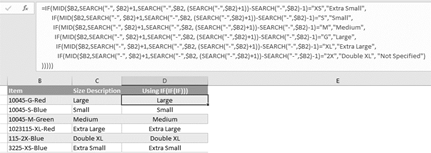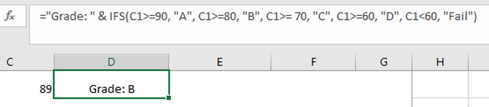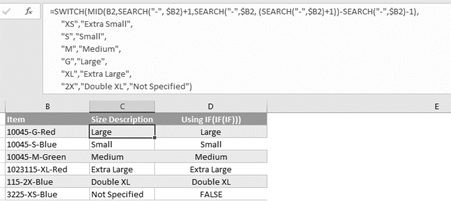The logical function IFS is available if you are an Office 365 subscriber and have the latest version of Office installed on your PC or you are using Excel Online. It is also available in Excel Mobile and in Excel for Android phones and tablets.

The IF function is one of the most commonly used logical functions in Excel, and using IF inside IF (nested IF functions) has been a common practice in Excel, but it can be challenging or confusing at times.

Here is an example of cluttered/ nested Ifs:The new IFS function help specify a series of conditions. IFS gives you an alternative to using a series of nested IF functions, when you have more than one condition that you want to test to find a corresponding result. The IFS function checks whether one or more conditions are met and returns a value that corresponds to the first TRUE condition.

The advantage of using the new IFS functions is that you can specify a series of conditions in a single function. Each condition is followed by the result that will be used if the condition is true—making it very straightforward to create and read the formula afterward.

Here is the syntax:

IFS(logical_test1, value_if_true1, [logical_test2, value_if_true2], [logical_test3, value_if_true3],…)

Because Excel functions are limited to 254 parameters, you can use up to 127 pairs of condition and result arguments in this IFS function.

For example, let’s say you want to get the grade letter for a given score on a test. Using the IFS function, it might be something like this:

=IFS(C1>=90, “A”, C1>=80, “B”, C1>= 70, “C”, C1>=60, “D”, C1<60, “Fail”)This can be read as, if the grade in C1 is greater than or equal to 90, it’s an A. Otherwise, if it’s greater than or equal to 80, it’s a B. Otherwise, if it’s greater than or equal to 70, it’s a C and so on. It’s pretty easy to write it this way and it’s also straightforward to read and understand what’s going on.

Note:

The SWITCH function is also a powerful logical function and can handle multiple conditions. What makes it different is that rather than specifying a series of conditional statements, you specify an expression and a series of values and results. The values are compared to the expression, and when the first exact match is found, the corresponding result is applied to the cell. You can also specify a “default” result that will be returned if none of the values are an exact match for the expression. The advantage of the SWITCH function is that you can avoid repeating the expression over and over, which sometimes happens in nested IF formulas.

In the example below, the first part of the formula extracts the size code (i.e. XS, M and G) from the middle of the item in column B. It’s rather long, so it’s nice that SWITCH only needs it to be written once and it can be compared to a list of values.

The example below can be explained as:

Extract the size code from the item in column B. If it equals “XS”, the result is “Extra Small.” Otherwise, if it equals “S”, the result is “Small” and so on. If there’s no match, the result is “Not Specified.”Again, because Excel functions are limited to 254 arguments, you can use up to 126 pairs of value and result arguments in this SWITCH function.